# slant2range

Convert slant range to propagated range

Since R2022b

## Syntax

r = slant2range(sr,anht,tgtht)
r = slant2range(sr,anht,tgtht,Name=Value)
[r,el,k] = slant2range(sr,anht,tgtht,___)

## Description

example

r = slant2range(sr,anht,tgtht) returns the propagated range r between a target and sensor as a function of the true target slant range sr, antenna height anht, and target height tgtht. Propagated range is the actual curved path range caused by atmospheric refraction. Slant range is the geometric range between target and sensor. The range computation assumes a Curved Earth Model where the atmospheric model is the CRPL exponential reference atmosphere with a refractivity of 313 N-units and a refraction exponent (decay constant) of 0.143859/km. The exponential atmosphere models refraction for elevation angles greater than approximately 10 millirad (about 0.573 degrees) and heights above approximately 1 km.
r = slant2range(sr,anht,tgtht,Name=Value) specifies additional inputs using name-value pair arguments.

example

[r,el,k] = slant2range(sr,anht,tgtht,___) also returns the target elevation el and the effective earth radius factor k.If the outputs are returned as r = NaN, el = NaN, and k = 1, then the propagation path does not exist or cannot be computed with the specified sr, anht, and tgtht arguments.

## Examples

collapse all

Calculate the propagated range from a slant range of 100 km, antenna height of 1 km, and target height of 2 km. Use default parameter values.

R = slant2range(100000,1000,2000)
R = 1.0001e+05 

Compute the range between an antenna and a target. Start with a slant range of 250 km, an antenna height of 1 km, and target height of 5 km. Using the default 'Curved' Method, set an effective earth radius factor of 1.2.

Re = physconst('EarthRadius'); effactor = 1.2; R = slant2range(250000,1000,5000,'EffectiveEarthRadius',effactor*Re)
R = 2.5002e+05 

Calculate the propagated range and elevation angle of a target at a slant range of 300 km, an antenna height of 100 m, and target height of 5 km. Use the CRPL method and assume the surface refractivity is equal to 400 N-units. From the calculated propagated range and elevation angle, convert back to target height. Verify that the estimated target height from the range2height function matches the expected 5 km.

sr = 300000; ha = 100; ht = 5000; Ns = 400; rexp = refractionexp(Ns);

Calculate propagated range and elevation angle.

[R,el] = slant2range(sr,ha,ht,'Method','CRPL', ... 'SurfaceRefractivity',Ns,'RefractionExponent',rexp)
R = 3.0009e+05 
el = 0.1286 

Convert propagated range and elevation angle back to target height. Verify that calculated value matches 5000 meters.

htest = range2height(R,ha,el,'Method','CRPL', ..., 'SurfaceRefractivity',Ns,'RefractionExponent',rexp)
htest = 5.0000e+03 

Compare the effective Earth radius factors calculated from the CRPL, the average radius of curvature, and 4/3 Earth models. Assume the slant range is 100000 m, the antenna heights range from 1 to 10 km, and the target is on the surface at zero altitude.

sr = 100000; ha = linspace(1,10,50).*1000; ht = 200; [~,kAvgCurv] = effearthradius(sr,ha,ht); [~,~,kCRPL] = slant2range(sr,ha,ht,'Method','CRPL');

Plot the effective earth radius factor as a function of antenna height.

plot(ha*1e-3,kCRPL) hold on plot(ha*1e-3,kAvgCurv) yline(4/3,'--k') grid on legend('CRPL','Average Curvature','4/3 Earth') ylabel('Effective Earth radius factor k') xlabel('Antenna height (km)')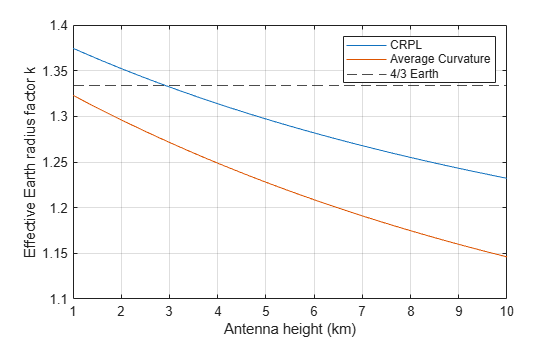## Input Arguments

collapse all

True slant range, specified as a scalar or length-M real-valued row vector. If sr is a vector, it must have the same size as the other vector input arguments, anht and tgtht. Units are in meters.

Example: 5000.0

Data Types: double

Sensor height in meters, specified as a nonnegative real-valued scalar or vector. If anht is a vector, it must have the same size as the other vector input arguments of slant2range. Heights are referenced to the ground.

Data Types: double

Target height in meters, specified as a nonnegative real-valued scalar or vector. If tgtht is a vector, it must have the same size as the other vector input arguments of slant2range. Heights are referenced to the ground.

Data Types: double

### Name-Value Arguments

Specify optional pairs of arguments as Name1=Value1,...,NameN=ValueN, where Name is the argument name and Value is the corresponding value. Name-value arguments must appear after other arguments, but the order of the pairs does not matter.

Before R2021a, use commas to separate each name and value, and enclose Name in quotes.

Example: Method="CRPL",SurfaceRefractivity=300,RefractionExponent=0.15

Earth model used for computation, specified as "Curved" or "CPRL".

Data Types: char | string

Effective Earth radius, specified as a positive scalar. If this argument is not specified, slant2range calculates the effective Earth radius using a refractivity gradient of –39 × 10–9 N-units/meter, which results in approximately 4/3 of the real Earth radius. Units are in meters.

#### Dependencies

To enable this argument, set the Method name-value pair argument to "Curved".

Data Types: double

Surface refractivity in N-units, specified as a nonnegative scalar. The surface refractivity is a parameter of the CRPL Exponential Reference Atmosphere Model used by slant2range. This quantity is dimensionless.

#### Dependencies

To enable this argument, set the Method name-value pair argument to "CRPL".

Data Types: double

Refraction exponent, specified as a nonnegative scalar. The refraction exponent is a parameter of the CRPL Exponential Reference Atmosphere Model used by slant2range. This quantity is dimensionless.

#### Dependencies

To enable this argument, set the Method name-value pair argument to "CRPL".

Data Types: double

Maximum number of iterations for the CRPL method, specified as a nonnegative integer. This input acts as a safeguard to preempt long iterative calculations.

If MaxNumIterations is set to 0, slant2range performs a faster but less accurate non-iterative CRPL calculation. The non-iterative calculation has a maximum height error of 0.056388 m (0.185 ft) at a target height of 30,480 m (100,000 ft) and an elevation angle of 0. The height error for the non-iterative method decreases with decreasing target height and increasing elevation angle. This quantity is dimensionless.

#### Dependencies

To enable this argument, set the Method name-value pair argument to "CRPL".

Data Types: double

Numerical tolerance for the CRPL method, specified as a positive scalar. The iterative process terminates when the numerical tolerance is achieved.

#### Dependencies

To enable this argument, set the Method name-value pair argument to "CRPL" and set the MaxNumIterations name-value pair argument to be greater than 0. This quantity is dimensionless.

Data Types: double

## Output Arguments

collapse all

Target range, returned as a scalar or real-valued length-M row vector. If r is a vector, it has the same size as the vector input arguments of slant2range. Units are in meters.

Data Types: double

Elevation angle, returned as a scalar or real-valued length-M row vector. Units are in degrees.

Data Types: double

Effective earth radius factor, returned as a scalar or real-valued length-M row vector. This quantity is dimensionless.

Data Types: double

collapse all

### Curved Earth Model

The fact that the index of refraction of air depends on height can be treated approximately by using an effective Earth's radius larger than the actual value.

Given the effective Earth's radius R0, the antenna height ha, and the initial elevation angle θ0, the model relates the target height hT and the slant range RT by

${\left({R}_{0}+{h}_{T}\right)}^{2}={\left({R}_{0}+{h}_{a}\right)}^{2}+{R}_{T}^{2}+2{R}_{T}\left({R}_{0}+{h}_{a}\right)\mathrm{sin}{\theta }_{0},$

so knowing one of those magnitudes enables you to compute the other. In particular,

${h}_{T}=\sqrt{{\left({R}_{0}+{h}_{a}\right)}^{2}+{R}_{T}^{2}+2{R}_{T}\left({R}_{0}+{h}_{a}\right)\mathrm{sin}{\theta }_{0}}-{R}_{0}.$

The actual range R is equal to the slant range. The true elevation angle θT is equal to the initial elevation angle.

To compute the ground range G, use

$G={R}_{0}\varphi ={R}_{0}\mathrm{arcsin}\frac{{R}_{T}\mathrm{cos}{\theta }_{0}}{{R}_{0}+{h}_{T}}.$A standard propagation model uses an effective Earth's radius that is 4/3 times the actual value. This model has two major limitations:

1. The model implies a value for the index of refraction near the Earth's surface that is valid only for certain areas and at certain times of the year. To mitigate this limitation, use an effective Earth's radius based on the near-surface refractivity value.

2. The model implies a value for the gradient of the index of refraction that is unrealistically low at heights of around 8 km. To partially mitigate this limitation, use an effective Earth's radius based on the platform altitudes.

For more information, see effearthradius.

### CRPL Exponential Reference Atmosphere Model

Atmospheric refraction evidences itself as a deviation in an electromagnetic ray from a straight line due to variation in air density as a function of height. The Central Radio Propagation Laboratory (CRPL) exponential reference atmosphere model treats refraction effects by assuming that the index of refraction n(h) and the refractivity N decay exponentially with height. The model defines

$N=\left(n\left(h\right)-1\right)×{10}^{6}={N}_{\text{s}}{e}^{-{R}_{\text{exp}}h},$

where Ns is the atmospheric refractivity value (in units of 10–6) at the surface of the earth, Rexp is the decay constant, and h is the height above the surface in kilometers. Thus

$n\left(h\right)=1+\left({N}_{\text{s}}\text{\hspace{0.17em}}×{10}^{-6}\right){e}^{-{R}_{\text{exp}}h}.$

The default value of Ns is 313 N-units and can be modified using the SurfaceRefractivity name-value argument in functions that accept it. The default value of Rexp is 0.143859 km–1 and can be modified using the RefractionExponent name-value argument in functions that accept it.

### CRPL Model Geometry

When the refractivity of air is incorporated into the curved Earth model, the ray paths do not follow a straight line but curve downward. (This statement assumes standard atmospheric propagation and nonnegative elevation angles.) The true elevation angleis different from the initial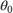. The actual range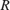, which is the distance along the curved path, is different from the slant range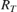.

Given the Earth's radius, the antenna height, the initial elevation angle, and the height-dependent index of refraction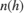with value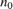at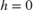, the modified model relates the target height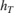and the actual rangebyWhen Method is specified as "CRPL", the integral is solved usingfrom CRPL Exponential Reference Atmosphere Model.

To compute the ground range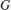, use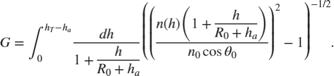### N-units

N-units are a convenient way to express the index of refraction. Because the index of refraction is very close to unity, N-units express just the deviation from unity. The refractivity N in N-units is related to the index of refraction n by

$N=\left(n-1\right)×{10}^{6}\text{.}$

For example, an index of refraction of 1.000313 becomes 313 in N-units. N-units are dimensionless.

 Barton, David K. Radar Equations for Modern Radar. Norwood, MA: Artech House, 2013.

 Bean, B.R., and G.D. Thayer. "Central Radio Propagation Laboratory Exponential Reference Atmosphere." Journal of Research of the National Bureau of Standards, Section D: Radio Propagation 63D, no. 3 (November 1959): 315. https://doi.org/10.6028/jres.063D.031.

 Blake, Lamont V. "Ray Height Computation for a Continuous Nonlinear Atmospheric Refractive-Index Profile." Radio Science 3, no. 1 (January 1968): 85–92. https://doi.org/10.1002/rds19683185.